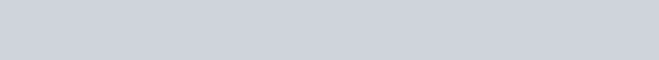# Python zip() function

Filed Under: Python

Good day learners, hope that you are doing well. We discussed about Python Modulo in our previous tutorial. In this tutorial we will learn about Python zip function.

## Python zip

If you are a regular computer user, you should have used .zip file extension. Do you know what it is? Basically, .zip is a container itself. It holds the real file inside.

Similarly, Python zip is a container that holds real data inside. Python zip function takes iterable elements as input, and returns iterator. If Python zip function gets no iterable elements, it returns an empty iterator.

### Python zip function example

Let’s look at a simple python zip function example. In previous section, we told about arguments taken by Python zip and what it returns. So by passing no argument, zip returns an empty iterator.

However, if we pass two iterable object of same lengths, then an iterable of python tuples will be returned where each element of the tuple will be from those iterable lists.

Python zip function is mainly used to combining data of two iterable elements together. See the following code.

``````
test = zip()

# referring a zip class
print('The type of an empty zip : ', type(test))

list1 = ['Alpha', 'Beta', 'Gamma', 'Sigma']
list2 = ['one', 'two', 'three', 'six']

test = zip(list1, list2)  # zip the values

print('\nPrinting the values of zip')
for values in test:
print(values)  # print each tuples

``````

So the output of the above python zip example program will be:

``````
The type of an empty zip :  <class 'zip'>

Print the values of zip
('Alpha', 'one')
('Beta', 'two')
('Gamma', 'three')
('Sigma', 'six')
``````

Notice the output of python type function call, so zip function returns instance of zip python class.

### Python zip example with iterable elements of different lengths

Now, what will happen if we try to zip two or more iterable elements? Well, In this case, the Python zip function will add items up to the lowest possible index of those given iterable elements.

That means the count of tuples will be same as the lowest length of the given iterable element. The following example will help you understand this.

``````
# list of 4 elements
list1 = ['Alpha', 'Beta', 'Gamma', 'Sigma']
# list of 5 elements
list2 = ['one', 'two', 'three', 'six', 'five']
# list of 3 elments
list3 = [1, 2, 3]

test = zip(list1, list2, list3)  # zip the values
cnt = 0

print('\nPrinting the values of zip')
for values in test:
print(values)  # print each tuples
cnt+=1

print('Zip file contains ', cnt, 'elements.');

``````

So the output of this code will be

``````
Printing the values of zip
('Alpha', 'one', 1)
('Beta', 'two', 2)
('Gamma', 'three', 3)
Zip file contains  3 elements.
``````

### Python extract zip

We can also extract data from the Python zip function. To extract zip, we have to use the same zip() function. But we have add an asterisk(*) in front of that list you get from zipped variable.

You can use list() function to get a list from zipped variable. However, this will return a number of tuple. The number will differ according to the number of arguments that the zip function took to zip the data. See the following code to understand.

``````
list1 = ['Alpha', 'Beta', 'Gamma', 'Sigma']
list2 = ['one', 'two', 'three', 'six']

test = zip(list1, list2)  # zip the values

testList = list(test)

a, b = zip( *testList )
print('The first list was ', list(a));
print('The second list was ', list(b));

``````

And the output will beNote that if the initial lists are of different length, then you won’t get the original list back. For example, if `list2 = ['one', 'two', 'three']` in above program, then output will be like below.

``````
The first list was  ['Alpha', 'Beta', 'Gamma']
The second list was  ['one', 'two', 'three']
``````

That’s all for Python zip function. Hope that you have learnt well. For further query please use the comment box.

Reference: Official Documentation

1.Taha AIT says:

Nice especially for dezipping

2.krishna says:

Good, very good..
Keep up.
The nice work.

3.Roshan Raj says:

Great Explanation with clear examples, helped me a lot in understanding a code which was available online…

4.Vimal Mahi says:

Nice explanation. Simple, concise & clear.

Thanks mate!